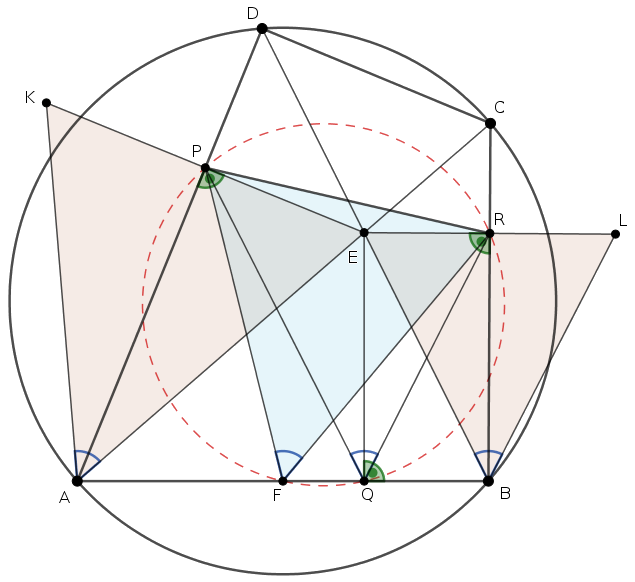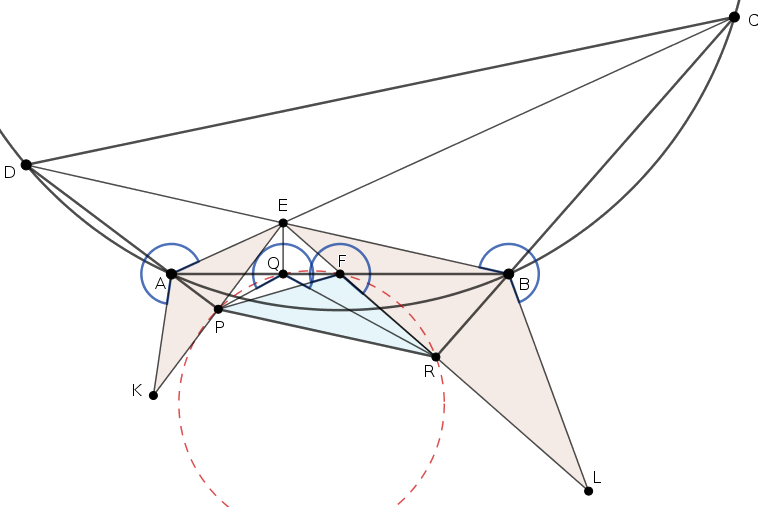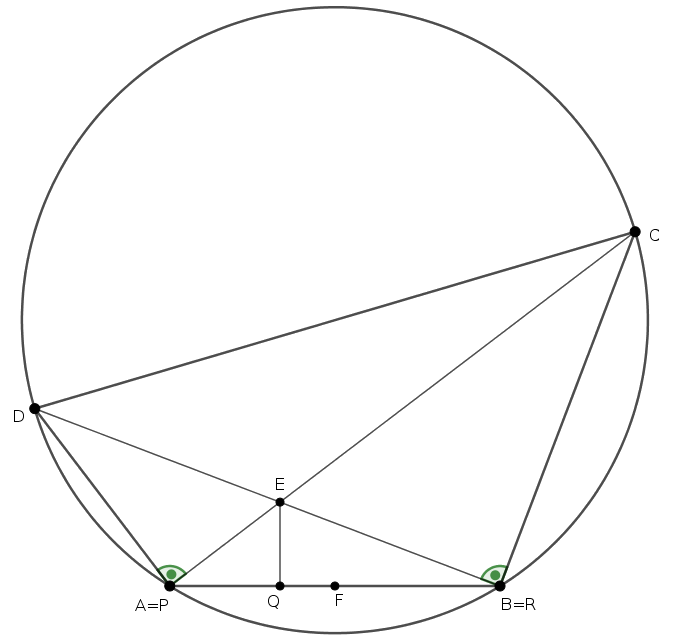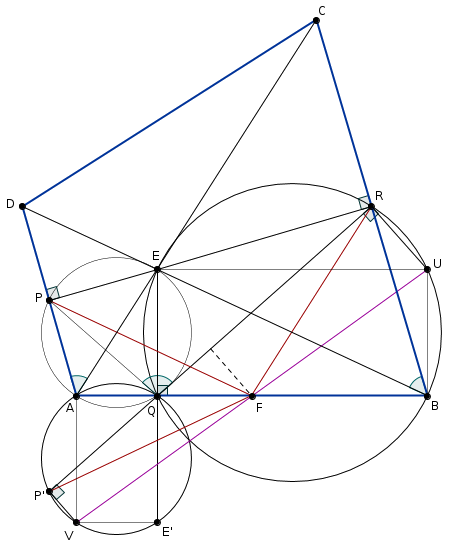Mathematical and Physical Journal
for High Schools
Issued by the MATFUND Foundation
 Already signed up? New to KöMaL?

#Problem A. 729. (September 2018)

A. 729. In a cyclic quadrilateral $\displaystyle ABCD$, the diagonals meet at point $\displaystyle E$, the midpoint of side $\displaystyle AB$ is $\displaystyle F$, and the feet of perpendiculars from $\displaystyle E$ to the lines $\displaystyle DA$, $\displaystyle AB$, and $\displaystyle BC$ are $\displaystyle P$, $\displaystyle Q$, and $\displaystyle R$, respectively. Prove that the points $\displaystyle P$, $\displaystyle Q$, $\displaystyle R$, and $\displaystyle F$ are concyclic.

Proposed by Máté Weisz, Szeged

(7 pont)

Deadline expired on October 10, 2018.

Solution 1.

Lemma. Let $\displaystyle A_1A_2\ldots A_n$ and $\displaystyle B_1B_2\ldots B_n$ be similar polygons with the same orientation. For every $\displaystyle i$, let $\displaystyle C_i$ be the midpoint of the line segment $\displaystyle A_iB_i$. Then either the points $\displaystyle C_1,C_2,\ldots,C_n$ coincide, or the polygon $\displaystyle C_1C_2\ldots C_n$ is similar to $\displaystyle A_1A_2\ldots A_n$ and has the same orientation.

Proof: Place the points on the complex plane. Let $\displaystyle \varphi$ be the orientation preservin similarity that moves the polygon $\displaystyle A_1A_2\ldots A_n$ to $\displaystyle B_1B_2\ldots B_n$. That is, $\displaystyle \varphi$ is a linear function, $\displaystyle \varphi(z)=az+b$ with some complex numbers $\displaystyle a\ne0$ and $\displaystyle b$. The transformation $\displaystyle \psi(z)=\frac{z+\varphi(z)}{2}$ maps the points $\displaystyle A_1,\ldots,A_n$ to $\displaystyle C_1,\ldots,C_n$, respectively. If $\displaystyle \psi(z)$ is constant then $\displaystyle C_1=\ldots=C_n$. Otherwise $\displaystyle \psi$ is also an orentation preserving similarity.

Proof for the problem statement::

Let $\displaystyle \sphericalangle DAC = \alpha$. Consider first the case when $\displaystyle \alpha$ is acute.

Let the reflections of $\displaystyle E$ about lines $\displaystyle AD$ and $\displaystyle BC$ be $\displaystyle K$ and $\displaystyle L$, respectively. Then

$\displaystyle \sphericalangle KAP = \sphericalangle PAE = \sphericalangle DAC = \sphericalangle DBC = \sphericalangle EBR = \sphericalangle REL = \alpha.$

As can be seen the isosceles triangles $\displaystyle AEK$ and $\displaystyle BLE$ are similar and the have the same orientation as the quatrilateral $\displaystyle ABCD$.

By applying the lemma to these triangles, we conclude that the midpoints of $\displaystyle AB$, $\displaystyle KE$ and $\displaystyle EL$ form another similar triangle, so $\displaystyle \triangle AEK \sim \triangle BLE \sim \triangle FRP$, and hence

$\displaystyle \sphericalangle PFR = \sphericalangle KAE = 2\alpha.$By the right angles at $\displaystyle P,Q,R$, the quadrilaterals $\displaystyle AQEP$ and $\displaystyle BREQ$ are cyclic, so $\displaystyle \sphericalangle PQE = \sphericalangle PAE = \alpha$ and $\displaystyle \sphericalangle EQR = \sphericalangle EDP = \alpha$, therefore

$\displaystyle \sphericalangle PQR = 2\alpha.$

This shows that $\displaystyle \sphericalangle PFR =\sphericalangle PQR = 2\alpha$; and porves tha problem statement.

If $\displaystyle \alpha$ is obtuse then $\displaystyle 2\alpha>180^\circ$, and the triangles $\displaystyle AEK$ and $\displaystyle BLE$ have reversed orientation. The proof can be done in the same way, by replacing $\displaystyle 2\alpha$ with $\displaystyle 360^\circ-2\alpha$:

$\displaystyle PFR\sphericalangle = PQR\sphericalangle = 360^\circ-2\alpha.$(The case consideration can be avoided if we use oriented angles.)

Finally, if $\displaystyle \alpha$ is a right angle then $\displaystyle P=A$ and $\displaystyle Q=B$; the points $\displaystyle P,F,Q,R$ are collinear.(In this case the statement is inaccurate; the circle $\displaystyle PQRF$ generates to a line.)

Solution 2. (outline).

Here we present the solution in the case when $\displaystyle \sphericalangle DAC$ is acute.

In the cyclic quardilaterals $\displaystyle AQEP$, $\displaystyle ABCD$ and $\displaystyle BREQ$ we have $\displaystyle \sphericalangle PQE = \sphericalangle DAC = \sphericalangle DBC = \sphericalangle EQR$, so $\displaystyle QE$ bisects $\displaystyle \sphericalangle PQR$; the line $\displaystyle AQB$ is the external angle bisectior of $\displaystyle \sphericalangle PQR$.

In any triangle, an external angle bisector and the perpendicular bisector of the opposite side meet on the circumcircle, so it suffices to proof that in triangle $\displaystyle PQR$, the point $\displaystyle F$ lies on the perpendicular bisector of $\displaystyle PR$, or equivalently, $\displaystyle FP=FR$.

Let $\displaystyle P'$ and $\displaystyle E'$ be the reflections of $\displaystyle P$ and $\displaystyle E$ about line $\displaystyle AQB$. The points $\displaystyle P',Q,R$ are collienar and $\displaystyle FP=FP'$, it is enough to proof $\displaystyle FP'=FR$.On the circles $\displaystyle BREQ$ and $\displaystyle AP'E'Q$, let $\displaystyle U$ and $\displaystyle V$ be the points opposite to $\displaystyle Q$, respectively. We claim that $\displaystyle F$ is the midpoint of $\displaystyle UV$. Notice that $\displaystyle AVE'Q$ and $\displaystyle BUEQ$ are rectangles, so

$\displaystyle \overrightarrow{QU}+\overrightarrow{QV} = \big(\overrightarrow{QB}+\overrightarrow{QE}\big)+ \big(\overrightarrow{QA}+\overrightarrow{QE'}\big)= \overrightarrow{QA}+\overrightarrow{QB} = 2\overrightarrow{QF}.$

That proofs that $\displaystyle F$ bisects $\displaystyle UV$.

Now consider the trapezoid $\displaystyle RP'VU$, that has right abgles at $\displaystyle R$ and$\displaystyle P'$. $\displaystyle F$ is the midpoint of $\displaystyle UV$, so $\displaystyle F$ lies on the midline of the trapezoid that is the perpendicluar bisector of $\displaystyle P'R$. Hence, $\displaystyle FP'=FR$, done.

### Statistics:

 12 students sent a solution. 7 points: Kerekes Anna, Márton Dénes, Milan Haiman, Schrettner Jakab, Shuborno Das, Szabó 417 Dávid, Szabó Kristóf, Weisz Máté. 6 points: Pituk Gábor. 4 points: 1 student. Unfair, not evaluated: 1 solutions. Not shown because of missing birth date or parental permission: 1 solutions.

Problems in Mathematics of KöMaL, September 2018